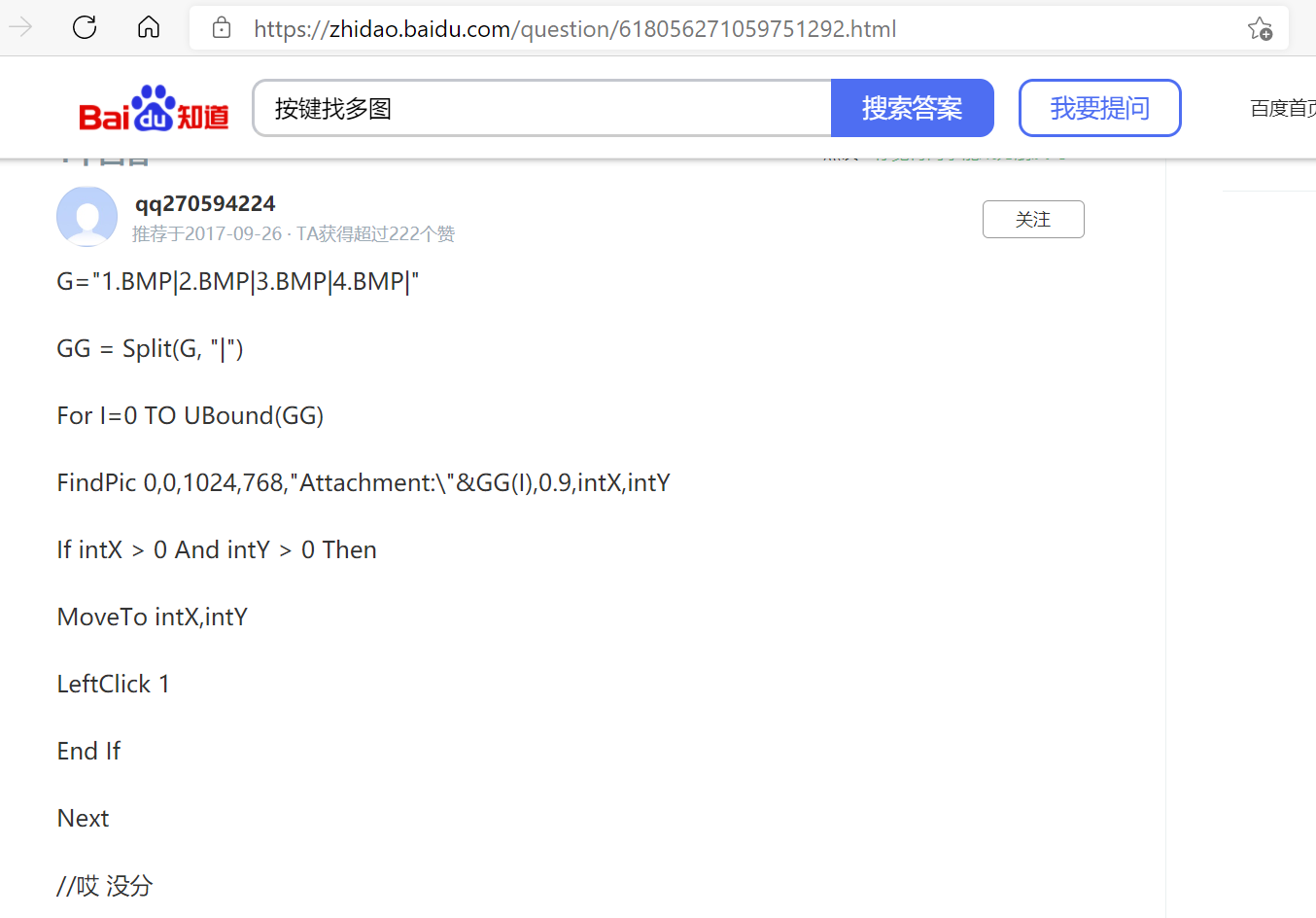## 按键精灵找多图的代码实现|按下标顺序找到一组图中的任意一张，找到后鼠标移动到坐标位置，并返回boolean“True“，找不到则返回“False“_按键精灵找多图任意一张点击-程序员宅基地``````Function countSubStr(string1,string2)
'查找string2在string1中出现的次数
start = 1
length = 1
countSubStr = 0
While start < len(string1) + 1
str = Left(string1, length)
If Instr(start, str, string2)>0 Then
countSubStr = countSubStr + 1
End If
start = start + 1
length = length + 1
Wend
End Function
Function locationPic(x1,y1,x2,y2,picName)
'查找图片，找到后鼠标移动到坐标
'查找区域为左上坐标x1,y1到右下坐标x2,y2
'返回boolean"True",否则返回"False",true=-1,false=0
'默认附件路径
FindPic x1, y1, x2, y2, "Attachment:\" & picName & ".bmp", 0.8, tempX, tempY
If tempX > 0 And tempY > 0 Then
MoveTo tempX, tempY
locationPic = True
Else
locationPic = False
End If
End Function
Function findMultiPics(x1,y1,x2,y2,pics)
'找多图,结合循环和判断语句可以有更多玩法，
'返回值类型为boolean,true=-1,false=0
'查找区域为左上坐标x1,y1到右下坐标x2,y2
'pics可以是单图名称，也可以用 | 符号连接的多个图片名
'找多图时按照下标顺序查找，找到后返回True，不再继续查找
'默认附件路径，在函数locationPic()中设置
separation = "|"
picArr = split(pics, separation)
cntSS = countSubStr(pics, separation)
If cntSS = 0 Then
findMultiPics = locationPic(x1, y1, x2, y2, pics)
Else
picCnt = 0
While picCnt <= cntSS
findMultiPics = locationPic(x1, y1, x2, y2, picArr(picCnt))
If findMultiPics = True Then
Goto ExitWhile
End If
picCnt = picCnt + 1
Wend
Rem ExitWhile
End If
End Function
Function loopFindMultiPics(x1,y1,x2,y2,pics)
'增强版找多图,找不到目标图片就一直找，直到找到为止
'返回值类型为boolean,true=-1,false=0
'查找区域为左上坐标x1,y1到右下坐标x2,y2
'pics可以是单图名称，也可以用 | 符号连接的多个图片名
'找多图时按照下标顺序查找，找到后返回True，不再继续查找
'默认附件路径，在函数locationPic()中设置
separation = "|"
picArr = split(pics, separation)
cntSS = countSubStr(pics, separation)
Do
If cntSS = 0 Then
loopFindMultiPics = locationPic(x1, y1, x2, y2, pics)
If loopFindMultiPics = True Then
Exit Do
End If
Else
picCnt=0
While picCnt <= cntSS
loopFindMultiPics = locationPic(x1, y1, x2, y2, picArr(picCnt))
If loopFindMultiPics = True Then
Exit Do
End If
picCnt = picCnt + 1
Wend
End If
Loop
End Function
'想要返回偏移坐标可以调用tempX和tempY``````

log: 这里是2023年6月9日,更新一下代码,前面写的代码虽然能用,但是太ugly,已经转行做程序员半年了,所以尝试更新一下,嘿嘿

``````Option Explicit
Function count_sub_str(str1, str2)
'计算字符串 str2 在字符串 str1 中的出现次数，并将结果存储在 cnt 变量中
Dim cnt
Dim start_pos
cnt = 0
start_pos = 1
Do While InStr(start_pos, str1, str2, vbTextCompare) > 0
cnt = cnt + 1
start_pos = InStr(start_pos, str1, str2, vbTextCompare) + 1
Loop
count_sub_str = cnt
End Function
Function locate_pic(pos_x1, pos_y1, pos_x2, pos_y2, pic_name, ByRef found_x, ByRef found_y)
'查找图片，找到后鼠标移动到坐标
'查找区域为左上坐标 pos_x1, pos_y1 到右下坐标 pos_x2, pos_y2
'返回 Boolean "True" 和 found_x、found_y 的值，True=-1，False=0
'默认附件路径
Dim ret
ret = False
FindPic pos_x1, pos_y1, pos_x2, pos_y2, "Attachment:" & pic_name & ".bmp", 0.8, found_x, found_y
If found_x > 0 And found_y > 0 Then
MoveTo found_x, found_y
ret = True
Else
ret = False
End If
locate_pic = ret
End Function
Function find_and_move_to_pic(pos_x1, pos_y1, pos_x2, pos_y2, pic_names, ByRef found_x, ByRef found_y)
'在指定区域查找指定图片，找到后移动鼠标至图片位置
'pic_names 可以是单个图片名称，也可以用 | 符号连接的多个图片名
'默认附件路径，在函数 location_pic() 中设置
Dim found
Dim separation
Dim pic_arr
found = False ' 定义返回值
separation = "|"
pic_arr = Split(pic_names, separation)
Dim cnt_ss
cnt_ss = count_sub_str(pic_names, separation)
Do
If cnt_ss = 0 Then
found = locate_pic(pos_x1, pos_y1, pos_x2, pos_y2, pic_names, found_x, found_y)
If found = True Then
MsgBox "找到目标图片！"
'使用 found_x 和 found_y 进行操作
Exit Do
End If
Else
Dim pic_cnt
pic_cnt = 0
While pic_cnt <= cnt_ss
found = locate_pic(pos_x1, pos_y1, pos_x2, pos_y2, pic_arr(pic_cnt), found_x, found_y)
If found = True Then
MsgBox "找到目标图片！"
'使用 found_x 和 found_y 进行操作
Exit Do
End If
pic_cnt = pic_cnt + 1
Wend
End If
Loop
If found = False Then
MsgBox "未找到目标图片！"
End If
find_and_move_to_pic = found
End Function
Function loop_find_and_move_to_pic(pos_x1, pos_y1, pos_x2, pos_y2, pic_names)
'在指定区域查找多张指定图片，找到后移动鼠标至图片位置
'pic_names 可以是单个图片名称，也可以用 | 符号连接的多个图片名
'默认附件路径，在函数 location_pic() 中设置
Dim found
Dim found_x, found_y
Dim looptimes
Dim MAX_TIMES
found = False
looptimes = 0
MAX_TIMES = 1000 ' 手动指定一下最大循环次数,防止死循环
Do
found = find_and_move_to_pic(pos_x1, pos_y1, pos_x2, pos_y2, pic_names, found_x, found_y)
If found = True And looptimes < MAX_TIMES Then
MsgBox "找到目标图片！"
'使用 found_x 和 found_y 进行操作
Exit Do
Else
looptimes = looptimes + 1
End If
Loop
If found = False Then
MsgBox "未找到目标图片！"
End If
loop_find_and_move_to_pic = found
End Function
``````

### 字符串模式匹配改进算法(KMP算法)-程序员宅基地

KMP算法是一种用于字符串匹配的算法,这个算法的高效之处在于当在某个位置匹配不成功的时候可以根据之前的匹配结果从模式字符串的另一个位置开始,而不必从头开始匹配字符串.因此这个算法的关键在于,当某个位置的匹配不成功的时候,应该从模式字符串的哪一个位置开始新的比较.假设这个值存放在一个next数组中,其中next数组中的元素满足这个条件:next[j] = k,表示的是当模式字符串中的第j + 1个(

### 三步骤详解张正友标定法-程序员宅基地

1998年，张正友提出了基于二维平面靶标的标定方法，使用相机在不同角度下拍摄多幅平面靶标的图像，比如棋盘格的图像，然后通过对棋盘格的角点进行计算分析来求解相机的内外参数。第一步：对每一幅图像得到一个映射矩阵（单应矩阵）H一个二维点..._张正友标定

### 程序员自我修养之长篇连载_main内存地址08048000-程序员宅基地

...不定期更新_main内存地址08048000

### 哈希表在Top-k问题中的应用--字符串_如何从哈希表中统计top k-程序员宅基地

1、介绍 数组的特点是：寻址容易，插入和删除困难； 链表的特点是：寻址困难，插入和删除容易。 这个世界上有没有一种能够综合两者优点的，既寻址容易又插入和删除容易的数据结构？Yes，它就是Hash表。2、哈希散列方法 （1）除留取余法 （2）平方散列法 （3）Fibonacci散列法3、哈希表使_如何从哈希表中统计top k

### The SplFileObject class_splfileobject类路径-程序员宅基地

SplFileObject类为文件提供了一个面向对象接口.http://php.net/manual/zh/class.splfileobject.php_splfileobject类路径

### AeroSpike的python压测代码_aerospike python-程序员宅基地

aerospike的压测，multiprocess，结果公布。_aerospike python